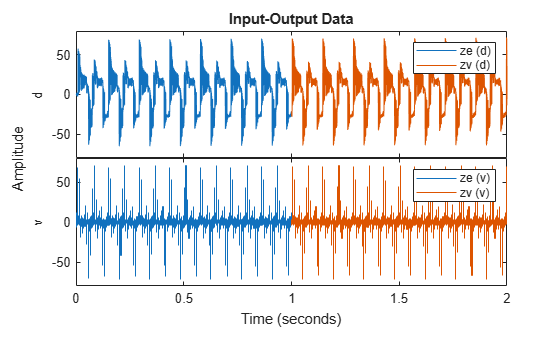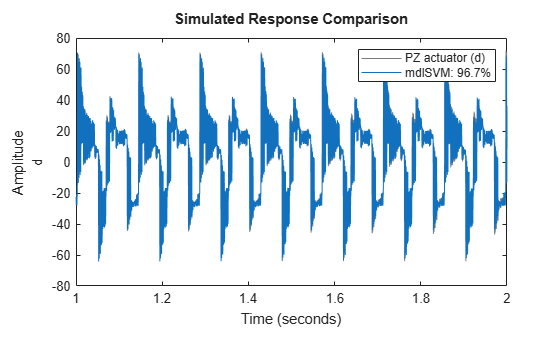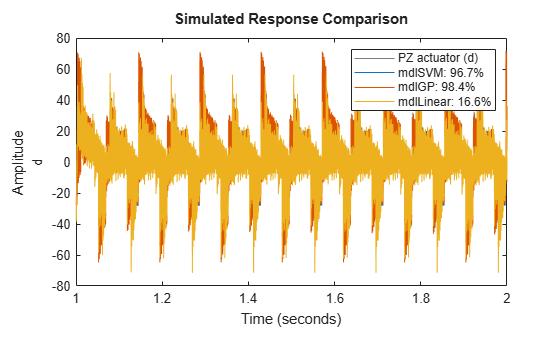# Piezoelectric Actuator Model Identification Using Machine Learning

This example shows identification of a piezoelectric actuator using machine-learning-based techniques that include support vector machines and Gaussian process functions.

### Experimental Setup

The data used in this example was provided by Prof. Micky Rakotondrabe (University of Toulouse, France) who performed the experiment in a laboratory in University of Franche-Comté at Besançon, France.

The experimental setup consists of a cantilevered piezoelectric actuator that is used in precise positioning applications. The actuator has two layers, namely, a piezoelectric layer that is made of lead zirconate titanate and a passive layer that is made of nickel. Piezoelectric actuators are used in several applications including high precision positioning and inkjet printing.

### Identification of Piezoelectric Actuators

Piezoelectric actuators suffer from several problems, including hysteresis, creep, and vibration. Models based on physical principles must take these characteristics into account. The identification process for piezoelectric actuator models usually involves estimating a mathematical model that combines a nonlinear component that characterizes the behavior of the hysteresis with a linear model that characerizes the linear dynamics of the actuator. This example uses two approaches that are based on machine learning to identify a black box model of a piezoelectric actuator. The example assumes that there is no prior knowledge about the dynamics of the actuator or the hysteresis nonlinearity.

### Input-Output Data

The input v is the voltage [V] applied to the actuator and the output d is the displacement of the actuator [$\mu \text{\hspace{0.17em}}$m]. The data set contains 10000 input-output samples corresponding to a sampling rate of 0.0002 s. This data will be used for all estimation and validation tasks carried out in this example.

```% load data load idPiezoElectricData.mat```

### Estimation and Validation Data

The input voltage applied to the piezoelectric actuator in this data set is a summation of sinusoidal signals with different frequencies. The data set is split into two parts, `ze` and `zv`, where the first 5000 samples are used for estimation (`ze`), and the remaining 5000 samples are used for validation (`zv`)

```ze = idPiezoElectricData(1:5000); % estimation data zv = idPiezoElectricData(5001:end); % validation data```

Plot the two data sets together to visually verify their time ranges

```figure; plot(ze, zv); legend('ze','zv');```### Identification Using Nonlinear ARX Model with Support Vector Machine Output Function

Configure a support vector machine (SVM) function to represent the nonlinearity in the system model.

Create the regressor set `Regressors` to use in the template `idnlarx` model.

```Vars = {'d', 'v'}; Lags = {1:20, 0:20}; Regressors = linearRegressor(Vars, Lags); ```

Configure the i`dSupportVectorMachine` nonlinearity object `NL`.

```NL = idSupportVectorMachine; NL.EpsilonMargin = 1e-3; NL.KernelScale = 30;```

Create a template `idnlarx` model `initialModel` that incorporates `Regressors` and `NL`. Then use `initialModel` to estimate an `NLARX` model `mdlSVM` from the data `ze`.

```initialModel = idnlarx(ze.OutputName, ze.InputName, Regressors, NL); mdlSVM = nlarx(ze, initialModel); ```

Visualize how the estimated model compares with the validation data.

`compare(zv,mdlSVM);`The legend in the figure shows a high fit accuracy for this model.

### Identification using Nonlinear ARX Model with Gaussian Process Output Function

Configure a Gaussian process (GP) function to represent the nonlinearity in the system model.

Create the regressor set `Regressors` to use in the template `idnlarx` model.

```% Create Regressors Vars = {'d','v'}; Lags = {1:5,0:5}; Regressors = linearRegressor(Vars, Lags);```

Configure the `idGaussianProcess` nonlinearity object `NL`.

`NL = idGaussianProcess; `

Create a template `idnlarx` model `initialModel` that incorporates `Regressors` and `NL`. Then use `initialModel` to estimate an `NLARX` model `mdlGP` from the data `ze`.

```initialModel = idnlarx(ze.OutputName, ze.InputName, Regressors, NL); mdlGP = nlarx(ze, initialModel);```

Visualize how the estimated model compares with the validation data.

`compare(zv,mdlGP);`The legend in the figure shows a high fit accuracy for this model.

### Compare Results for Nonlinear Models and Linear Model

Compare the identified models obtained using nonlinear ARX with support vector machines and Gaussian process output functions to an identified linear model obtained using the `ssest` command.

First set options for `ssest` using the option set `ssestOptions`. Then estimate a linear model `mdlLinear` using `ssest`.

```opt = ssestOptions; opt.EnforceStability = true; opt.Focus = 'simulation'; mdlLinear = ssest(ze,6,opt);```

Visualize how the three estimated models compare with the validation data.

```figure compare(zv,mdlSVM,mdlGP,mdlLinear);```The legend in the figure shows that the fit accuracy obtained using a linear model is significantly lower than the fit accuracies for both nonlinear ARX models with output functions based on machine learning.